Browse by Topic
Related Topics

# Mathematica Q&A Series: Surprises in Differentiation and Integration

Got questions about Mathematica? The Wolfram Blog has answers! We’ll regularly answer selected questions from users around the web. You can submit your question directly to the Q&A Team.

This week’s question comes from Kutha, a math lecturer:

Why doesn’t differentiating after integrating always return the original function?

Read below or watch this screencast for the answer (we recommend viewing it in full-screen mode):

The derivative of a definite integral with respect to its upper bound (with a constant lower bound) is equal to the integrand: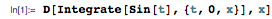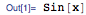This is a consequence of the fundamental theorem of calculus. (Note that sin(x) is equivalent to sin(t) up to renaming of the variable x or t.)

In complicated cases, it can appear that Mathematica is not giving back the original function: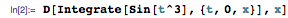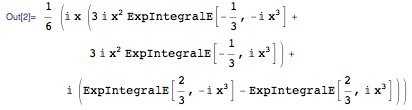However, this result is mathematically equivalent to the integrand sin(x3). You can often discover this fact by using Simplify or FullSimplify: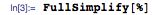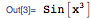That won’t necessarily work if you didn’t give the original integrand in its simplest form: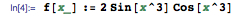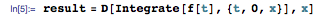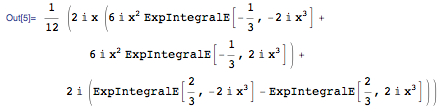Here, the simplified result is different from the integrand: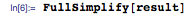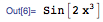In this case, you can try simplifying the integrand as well as the result: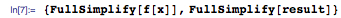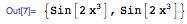Or take the easiest option and ask Mathematica to try to prove they are equal: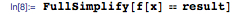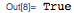Another calculus-related question concerns differentiation followed by indefinite integration. For this sequence of operations, the result is not necessarily mathematically equivalent to the original function, since an arbitrary constant of integration may be added (or subtracted):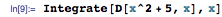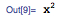In this case, it’s obvious that the original function and the result differ by the constant 5. In more complicated cases, it may not be immediately obvious what the differing constant of integration is: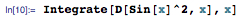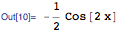Once again, you can use Simplify or FullSimplify to discover the difference: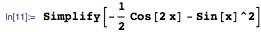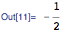For functions with branch cut discontinuities, indefinite integrals can be trickier: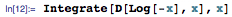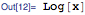Here, since log(x) and log(-x) have different branch cuts (from -∞ to 0 and from 0 to ∞, respectively), a consistent constant of integration isn’t possible. Instead, you get a “piecewise” constant of integration.

In the top half of the complex plane, log(x) and log(-x) differ by the constant i π: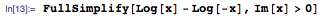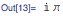While in the bottom half, they differ by –i π: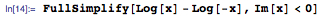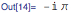You can avoid ambiguities related to branch cuts by specifying a definite integral from some point in the complex plane: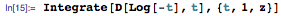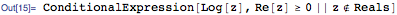The condition in the second argument of ConditionalExpression restricts the result to straight integration paths (from t = 1) that don’t intersect the branch cut running from z = -∞ to z = 0.

Depending on your starting point, the condition to avoid intersecting branch cuts can be quite complicated. For example, starting with t = 1 + i: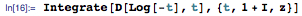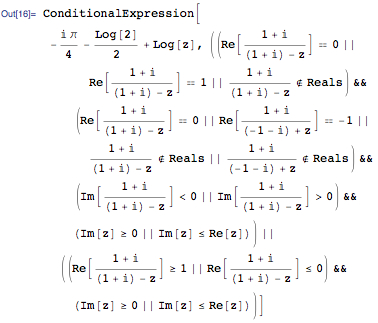That condition represents the following set of valid integration endpoints: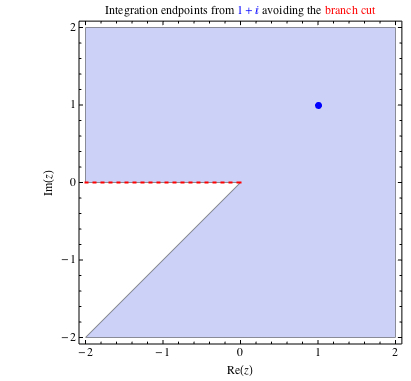Click here to download this post as a Computable Document Format (CDF) file, including the RegionPlot command used to generate the diagram above.

If you have a question you’d like answered in this blog, you can submit it to the Q&A Team. For daily bite-sized Mathematica tips, follow our @MathematicaTip Twitter feed.